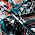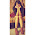## Jul 11, 2014

### 3D Tic Tac Toe Puzzle

Source: Shared by Alok Mittal (Cannan Partners)

Problem:
A 3x3 tic tac toe has 8 "winning lines" (3 horizontal, 3 vertical and 2 diagonals). How many "winning lines" does the 3x3x3 3D tictactoe have? There is a brute force solution, and then there is the aha! solution.

Update (23 Oct 2014)
Solution: Posted in comments by Anti, Taz, Javier, Shubham Gupta, Leela. Detailed solution and much more advanced problems in the document http://library.msri.org/books/Book42/files/golomb.pdf

#### 15 comments:

1.9 per face, 3 per edge, 1 per corner, divide by 2 for symmetry: (9*6 + 3*12 + 8)/2 --> 49

2.24 (8*3 Imagine 3 2D plates stacked horizontally)
24 (8*3 Imagine 3 2D plates stacked vertically)
4 (Diagonals of the cube)
Total of 52 winning lines

3.9 different cross-sections of dimension 1x3x3, each having 8 winning lines, plus 4 body diagonals. Hence, 9*8+4=76.

4.3 layers each of 3 rt angle faces. Each layer is a 3*3 tic tac toe
# of winning lines = 3*3*8 = 72

5.Total- 43
(24 - When 3 2D tic-tac planes are vertically stacked)
(15 - When 3 2D tic-tac planes are horizontally stacked minus number of winning lines already covered in previous case)
(4 - Diagonal winning lines)

6.For a simple 2 Dimensional Grid: 3 in x-direction + 3 along y +2 diagonals = 8 solutions

Extending same for 3 Dimensional Grid:

3*3 along x + 3*3along y +3*3 along z + 2*3 diagonals along xy + 2*3 diagonals along xz + 2*3 yz diagonals + 4 other diagonals = 49

7.I found this: http://library.msri.org/books/Book42/files/golomb.pdf

8.Is it 82 ?
72 + 4 + 6.

1.It won't be 82. Lots of repetition in 82.

9.Answer should be 49.
Top and bottom layer -
_______
|7|3|7|
|3|1|3|
|7|3|7|
_______

mid layer -
_______
|3|1|3|
|1|0|1|
|3|1|3|
_______

each matrix component tells about number of winning lines start/end at that cell.
sum = 98
ans = 98/2 = 49 (same line counted twice, from each cell it can start or end)

Winning lines will always be in direction along with x,y,z axis or 45 aligned from them .

10.Answer should be 49.
Top and bottom layer -
_______
|7|3|7|
|3|1|3|
|7|3|7|
_______

mid layer -
_______
|3|1|3|
|1|0|1|
|3|1|3|
_______

each matrix component tells about number of winning lines start/end at that cell.
sum = 98
ans = 98/2 = 49 (same line counted twice, from each cell it can start or end)

Winning lines will always be in direction along with x,y,z axis or 45 aligned from them .

11.76.

8 winning lines * 9 cross sections + 4 body diagonals

12.48 (8*6 faces) + 13 (26 points touching center and 2 will form a line with center) = 61 total

13.14.This is a very interesting question i must say.

One may compute the answer as 49 or 76 by two different approaches. Which one is correct, becomes a new question

I first computed 76 as the obvious answer: (8 winning lines per face) x (3 faces per direction) x (3 directions) + (4 Body diagonals) = 76

Then I looked at the approach of 49 as answer very easily illustrated in answer by Shubham Gupta which seems to be correct too.

So again 49 vs 76. Which is it. Take your pick!

Now think a bit carefully.
When one counts 76 winning lines, one considers many repetitions.
Focus on the front-top-left cube piece. When we count 76 winning lines, we count it three times.
Think it in the same way as a rubic cube has 27 pieces ( hence our tic-tac-toe has only 27 possible places to make a move) whereas it has 9*6 coloured stickers.
So when we consider 76 lines we are considering the stickers which is incorrect.

### Fraction Brainteaser

Source: Sent to me by Gaurav Sinha Problem: Siddhant writes a Maths test and correctly answers 5 out of 6 Arithmetic questions and 20...